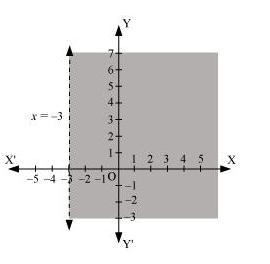# Solve the given inequality graphically in two-dimensional plane: x > –3

Question:

Solve the given inequality graphically in two-dimensional plane: x > –3

Solution:

The graphical representation of x = –3 is given as dotted line in the figure below. This line divides the xy-plane in two half planes.

Select a point (not on the line), which lies in one of the half planes, to determine whether the point satisfies the given inequality or not.

We select the point as (0, 0).

It is observed that,

0 > –3, which is true

Also, it is evident that any point on the line does not satisfy the given inequality.

Hence, every point on the right side of the line, x = –3 (excluding all the points on the line), determines the solution of the given inequality.

The solution region is represented by the shaded region as follows.